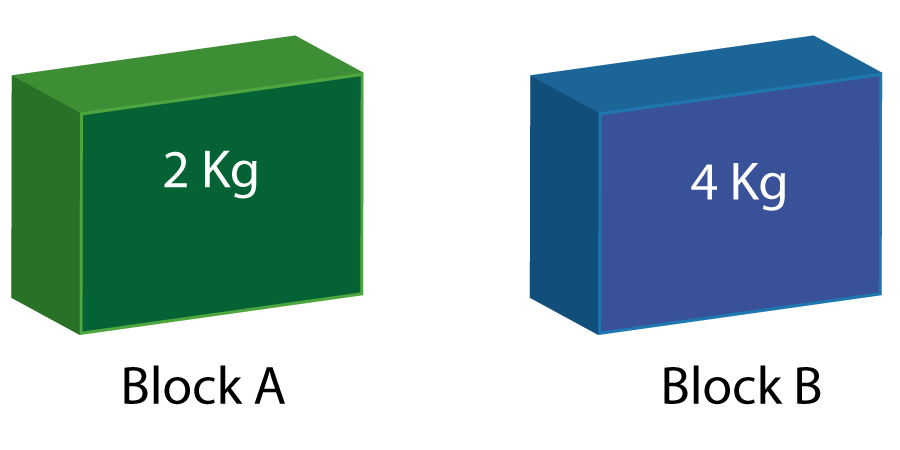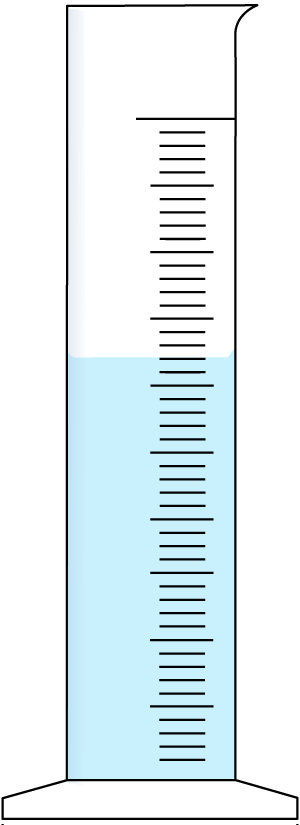Density, pressure, particles, heat transfer

1-4: These questions are about states of matter:
1. Which of these sentences is true?
• A). In a gas the particles have strong forces between them
• B) Liquids fill completely the container they are in
• C) The forces are strong between particles in a solid
• D) Particles in a liquid move faster than in a gas
Which of these descriptions matches which state of matter?
2. Particles are widely separated.
3. Does not have a fixed shape.
4. Strong bonds hold the particles firmly in place.

5. Which answer best describes the difference between these 2 blocks?• A) Block A has a larger volume than block B
• B) Block B is denser than block A
• C) Block A has more mass than block B
• D) Block A has more tightly packed particles than block B

6. What is the formula for density?

• A) Density = volume x mass
• B) Density = volume / mass
• C) Density = mass / volume
• D) Density =  ½ x mass x (volume)
7. Which of these calculations below is the right one to find the mass of water in the cylinder shown here?• A) (mass of cylinder) + (mass of water and cylinder)
• B) ( mass of cylinder and water) - (mass of water)
• C) (mass of cylinder) - (mass of water and cylinder)
• D) ( mass of cylinder and water) - (mass of cylinder)

8-10: What are the missing values in this table showing the density of various substances?

 Substance Mass (g) Volume(cm3) Density (g/cm3) 8 Wood 20 25 ? 500 50 0.8 1.25 9 Copper ? 324 4 0.25 25 36 9 10 Glass 400 ? 100 160 800 1000 2.5

11+12. Under a microscope, dust particles appear to move randomly around.

11. What is this effect called?

• A) random motion
• B) newtonian motion
• C) zero point motion
• D) brownian motion

12. What causes this motion?

• A) fast moving atoms / molecules colliding with the dust particles
• B) dust particles colliding with other dust particles
• C) gravity pulling on the dust particles
• D) random kinetic motion

13. A device for measuring temperature can be created by two wires made of different metals joined at the end. This is called...

• A) a thermometer
• B) a thermocouple
• C) a thermopile
• D) a thermocable